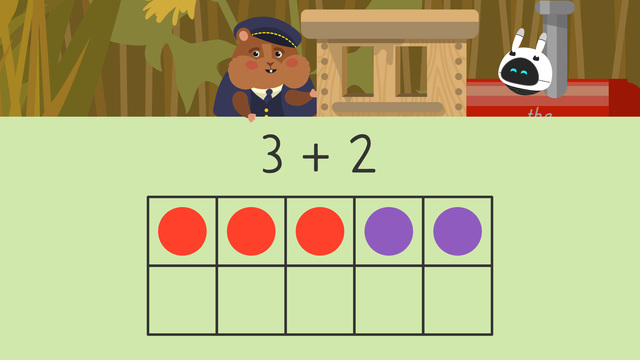# Using Ten Frames to AddRating

Ø 5.0 / 1 ratings

The authorsTeam Digital
CCSS.MATH.CONTENT.1.OA.C.6

## Basics on the topicUsing Ten Frames to Add

### In this Video on Addition with Ten Frames

The Hamtrack Express is almost ready for its first passengers. However, Conductor Squeaks just has to figure out how many train cars to add. In this video on ten frames addition, he determines the right number by using ten frames addition to 20. But where has Imani been this whole time?

### How to Use Ten Frames in Addition

Ten frames are charts with two equal rows of five. They have ten spaces in total. When we place counters in those spaces, we can see different numbers within ten. For example, the number six has six counters in a ten frame. Each box has one marker, or counter, and there are six in all.If we fill up the entire ten frame, it means we have a set of ten! When we see a full ten frame, we know the amount is ten right away without having to count!We can use a ten frame horizontally or vertically. It works the same both ways because the amount of spaces does not change.### Why Use Ten Frames in Addition?

Modeling addition using a ten frame helps children visualize the numbers you are adding together. Since each number is represented on the ten frame, it allows children to count out their answers.

Let’s use the example three plus two. Start by showing the first number, three, in counters on the ten frame.Then, show the second number, two, on the ten frame using counters again.Now, count ALL the filled spaces on the ten frame.There are five counters in all, so three train cars plus two train cars equals five train cars.• Look at the first number in the problem and put that many counters on the ten frame.

• Then, look at the second number and put that many more counters on.

• Finally, count all the filled spaces on the ten frame and write the answer.

• If a ten frame is full, you know you have ten without needing to count.

• If you fill up one ten frame, and still have more counters left, use another ten frame.

### Practice: Addition Using Ten Frames Worksheets

Have you practiced yet? On this website, you can also find ten frame addition worksheets and exercises.